Select Page

# Application of Integrals Maths 12 Science CBSE Solutions for MCQ in English

Application of Integrals Maths 12 Science CBSE Solutions for MCQ in English to enable students to get Solutions in a narrative video format for the specific question.

Expert Teacher provides Application of Integrals Maths 12 Science CBSE Solutions for MCQ through Video Solutions in English language. This video solution will be useful for students to understand how to write an answer in exam in order to score more marks. This teacher uses a narrative style for a question from Application of Integrals not only to explain the proper method of answering question, but deriving right answer too.

Please find the question below and view the Solution in a narrative video format.

Question:

Solution Video in English:

## Similar Questions from CBSE, 12th Science, Maths, Application of Integrals

Question 1 : Using the method of integration, find the area of the region bounded by the lines: 5x - 2y -10 = 0, x + y - 9 = 0 and 2x - 5y - 4 = 0. (View Answer Video)

Question 2 : Find the area of smaller region bounded by the ellipse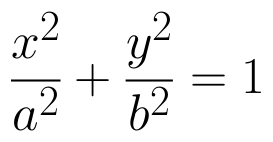and the line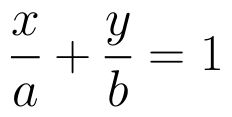. (View Answer Video)

Question 3 :

Find the area of the given curves and given lines: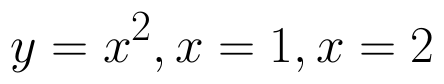and x-axis

Question 4 : Find the area bounded by the curve y = sin x between x = 0 and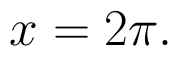(View Answer Video)

Question 5 : Using integration, find the area of the triangle ABC, where A is (2,3), B is (4,7) and C is (6,2). (View Answer Video)

### Application of Derivatives

Question 1 : A tank with a rectangular base and rectangular sides, open at the top is to be constructed so that its depth is 2m and volume is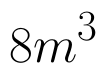If the building of tank costs Rs.70 per sq meter for the base and Rs.45 per sq meter for sides. What is the cost of least expensive? (View Answer Video)

Question 2 : The function f is a differentiable function and satisfies the functional equation f(x) + f(y) = f(x + y) – xy – 1for every pair x, y of real numbers. If f(1) = 1, then the number of integers n ≠ 1 for which f(n) = n is_______________. (View Answer Video)

Question 3 : The rate of change of the area of a circle with respect to its radius r at r = 6 cm is : (View Answer Video)

Question 4 : The normal at the point (1, 1) on the curve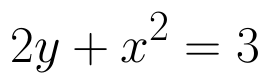is; (View Answer Video)

Question 5 : Find two positive numbers x  and y such that x+y=60 and is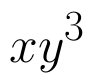maximum. (View Answer Video)

### Probability

Question 1 : Out of a group of 30 honest people, 20 always speak the truth. Two persons are selected at random from the group. Find the probability distribution of the number of selected persons who speak the truth. Also find the mean of the distribution. What values are described in this question?     (View Answer Video)

Question 2 : A bag contains 4 green and 6 white balls. Two balls are drawn one by one without replacement. If the second ball drawn is white, what is the probability that the first ball drawn is also white?   (View Answer Video)

Question 3 : A speaks truth in 60% of the cases, while B in 90% of cases. In what percent of cases are they likely to contradict each other in stating the same fact? In the cases of contradiction do you think, the statement B will carry more weight as he speaks truth in more number of cases than A?    (View Answer Video)

Question 4 : The probabilities of two students A and B coming to the school in time are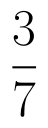and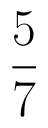respectively. Assuming that the events ' A coming in time ' and
' B coming in time ' are independent. Find the probability of only one. Write of them come in time atleast one advantage of coming to school in time.   (View Answer Video)

Question 5 : In a hockey match, both teams A and B scored same number of goals up to the end of the game, so to decide the winner, the referee asked both the captains to throw a die alternately and decided that the team, whose captain gets a six first, will be declared the winner. If the captain of the team A was asked to start, find their respective probabilities of winning the match and state whether the decision of the referee was fair or not.      (View Answer Video)

### Linear Programming

Question 1 : The objective function is maximum or minimum, which lies on the boundary of the feasible region. (View Answer Video)